APPENDIX

A Mathematical Model of a Psychosomatic System
Presented in the Cross-notation of
G. Spencer Brown’s Calculus of Indications

Imagine the initial physiological identity of a psychosomatic system to be that of a quantum of action existing at a point. This quantum’s spatial locality is plotted in real, spherical coordinates. In manifesting particulate behavior, the quantum takes on binary identity, i.e., it rotates in either a clockwise (-) or counterclockwise (+) manner. Whole integers or simple fractions (1/2, 1/4, …) provide the only accurate count of the particle’s rotations. This rotational identity of the quantum, now a particle, requires a temporal parameter -- the conjoint space-time aspect of identity being represented by Hamilton’s quaterions, which are complex numbers having real and imaginary components (i.e., based on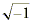). Active aspects of time give the system the freedom to change its rotational identity -- second and third orders of active time being represented by hypercomplex numbers beyond quaterions.

Imagine the initial psychological identity of a psychosomatic system to be that of a quantum of inaction existing at a counterpoint. This quantum’s counterspatial existence is plotted in real, hyperspherical coordinates. In manifesting active attributes, the quantum takes on binary identity, i.e., it adopts either conscious (-) or unconscious (+) states. Whole integers or simple fractions (1/2, 1/4, …) provide the only accurate count of the quantum’s logical operations of transit between states. This active identity of the quantum, now a psyche, requires a temporal parameter -- the conjoint counterspace-time aspect of identity being represented by counterquaterions, which are counterhypercomplex numbers having real and counterimaginary components (i.e., based on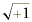). Active aspects of time give the system the freedom to change the order of logical-value of its identity, i.e., from 2-valued to m-valued -- second and third orders of active time being represented by counterhypercomplex numbers beyond counterquaterions.

Absent time in its 3-fold active aspect, absent space or counterspace, the psychosomatic system would be unable to re-order itself. Soma and psyche do not so much parallel one another, they mirror and exist in null-potential relationship, which is neither this or that, neither action or inaction, but nonaction. Anti-matter (self-duality/anti-self-duality) is psyche apprehended without advantage of the notions of counterspace and the active aspects of time.

Shifting the discussion now for simplicity’s sake (so as not to repeat the terminology in counterpoint) primarily to the spatial physiological modality, while implicitly recognizing the counterspatial psychological modality, we note that if spin above or below a certain threshold occurs, it would be impossible to count or quantize the event within the limited spacetime domain defined by this threshold. Thus, the identity of this system and its permissible changes are found within limits requiring both a relative zero (0) and (¥) mark. Both of these relative limits are set by linear-time, which provides a background reference state for interpreting the changing identity of the system. Rotations which are too fast or too slow to be registered lie outside the limited spacetime domain’s self-defining limits on sensory perception.

We have now provided the foundations for a mathematical model of a psychosomatic system built from quantum and relativistic principles. To interpret the mathematical model presented in Table 1, consider that the rotating point establishes its identity along a line by means of linear-time. This constitutes the first aspect of active time. In the second stage, this same line establishes its identity and the identity of the originating point over a curved surface through the action of cyclic time, the second aspect of active time. Finally, the third aspect of active time communicates the point-line-surface identity to a sphere.

The spatial expansion-contraction of information represented above as an idealized geometrical construct may be alternatively expressed using concepts from physics. Each quantum of activity defined to exist at a point, line, surface, or a sphere represents the realization of kinetic energy derived from an initial potential energy state. This conversion from potential into kinetic energy introduces a “free energy” into the domain which becomes manifest as coherent waves. These coherent waves, in turn, serve to communicate the scale interactivity which achieves the potential to kinetic energy conversion into the next higher level of the informational hierarchy.

It is through the third or hyparxical (“ableness to be”) aspect of active time that the psychosomatic system is able to complete its psychodynamic function of communicating its identity across both of its self-defining limits (0, ¥) with perfect efficiency. Thus, the fully-identified sphere exists as a concrete representation of infinity which has been fused with the originating point’s identity. Likewise, this same sphere simultaneously portrays a new point of identity whose localization serves to define a new larger-scale arena for informational processing. In the above discussion, we have defined this arena as a limited spacetime domain.

The 3-fold topological operations upon space and counterspace described by the three aspects of active time (linear, cyclic, and hyparxical) thus fulfill the requirements of functional integration and specificity. The simultaneity of this psychosomatic process may be summarized by: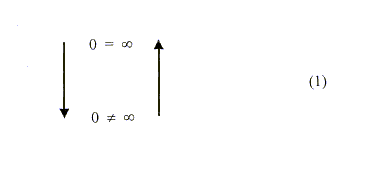which condenses the mathematical formalism given in Table 1. This formalism expresses a set of permissible operations upon the binary set of singularities (0, ¥). Mathematicians use the adjective “naked” with regard to such singularities because the term serves to express the concept that 0 and ¥ both define a limited spacetime domain, while existing outside of space and time.

In most physical problem solving, the zero and infinity states of the system are considered to represent “trivial” solutions. The present approach, in contrast, considers the zero and infinity states to designate singularities at the interface between limited spacetime domains. All the energy dynamics of critical and cooperative exchange processes are considered to take place at these singularities. If we proceed to split the foregoing expression for the psychosomatic system into its upper (0 = ¥) and lower (0 ¹ ¥) components, then map the intervening steps represented by the opposing arrows in (1), we will be able to discuss Table 1 in some detail.

From the state of pure consciousness-without-an-object, denoted by (1a), in which there is no differentiation of space and counterspace from time, we first obtain (2). The right-hand crosses (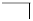) portray successive differentiations with respect to active time understood in their spatial physiological context, while the left-hand crosses
(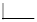) portray the same differentiations understood in their counterspatial psychological context. It is at this point that we introduce the real axes: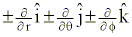and their complementary imaginary axes: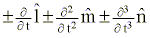to further categorize the fusion-localization process presented in (3).

It should be noted that the spatial del operator (±Ñ) is “informed” through the complementing roles of positive and negative temporal operations. Thus the spatial fusion of the identity of the singularity denoted by the 0-mark is simultaneously accompanied by the spatial localization of the identity of the singularity denoted by the ¥-mark. In the format of differentiable space and time, we may rewrite (3) as follows: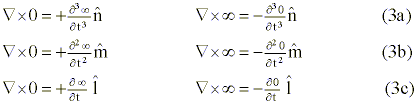Our next task consists of representing a specific (rotational) identity within the relative limits of 0 and ¥. We designate the state of mass-momentum balance in the system by the symbol F, and the state of mass-momentum imbalance by the symbol X. When the identity of the system remains locked within a particular spatial aspect (e.g., an equipotential surface), the mass-momentum field of that spatial aspect remains in a state of balance (F). But when the identity of the system is being communicated (such as from that of a curved surface to a sphere), then the curved surface will experience a mass-momentum imbalance designated by X. in (4).

Let us present (4) in its expanded format so we may elaborate upon the spatial expansion-contraction of information achieved through the parametersdefined below: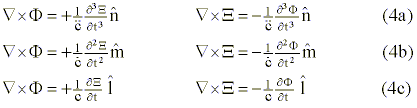The limiting velocity (c), limiting acceleration (), and limiting time rate of change of acceleration (), in (4c), (4b), and (4a), respectively, appear within the appropriate coherent wave equations where they initiate the critical phase transitions governing the potential Û kinetic energy conversion. All three of these limiting parameters serve as relative absolutes whose values are set by the zero- and infinity-marks defining the limited spacetime domain in question. These equations are written in Maxwellian form, which is most appropriate to the helix-coil transitions transpiring in the limited spacetime domain of the DNA molecule. These equations could also be written in Schrödinger form. The collapse, or spontaneous localization, of the wave function
[j (F, X)] is represented by reading the set of equations from (4a) through (4b) to (4c). Representation of the wave function’s recomposition, spontaneous fusion, requires reading the same set in the reverse direction. “Collapse” of the wave function is actually decomposition of the m-logically-valued supersymmetry relations underlying space and counterspace. Topological transformation from space to counterspace involves nonorientable supersymmetry conversion of the active temporal operators, which may be designated “crossover time” (Maharloka in Sanskrit).

If we disallow the interconnected potential Û kinetic energy conversion achieved through topological operations of active time, then we may write the baseline equation given in (1b) in Table 1. This forms the lower limit from which one begins to describe the nature of a psychosomatic system. This is equivalent to saying that a particular spatial identity defined with the aid of linear-time will remain transfixed and unable to transmute by means of the specific roles we have assigned to the three aspects of active time. In other words, a particular potential-to-kinetic energy conversion will remain an isolated event.

If 0 = ¥ represents our thesis, and 0 ¹ ¥ its antithesis, then the psychosomatic system stands as the compositional synthesis between the couplet of the equality expressed in (la) and the inequality expressed in (lb). The expression 0¹ ¥ is the defining characteristic of an isolated system reduced to its physiological aspect and processing information in a single-valued fashion. The expression 0 = ¥ is the defining characteristic of a self-referential psychosomatic system undergoing self-reentrant autocatalytic multiple scale interactions and processing information in a multivalued fashion.